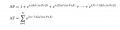# physics of beam steering directivity

#### yef smith

Joined Aug 2, 2020
398
Hello ,I have learned in class that by performing beam steering we decrease the effective area of the array and thus reduce the directivity.
In the array factor shown bellow.
beta=kdsin(theta)
so if we scan by 45 degrees we got an exponent coefficient.
but we take the absolute value of the array factor so this phase exponent is 1.
is there any mathematical proof where we could see that our directivity will reduce as the steering angle goe from 0 to 90 degree direction?
Thanks.